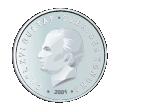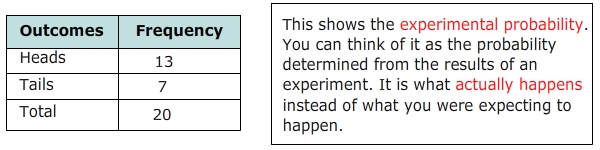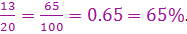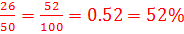# Theoretical vs. Experimental Probability

When asked about the probability of a coin landing on heads, you would probably answer that the chance is ½ or 50%.Imagine that you toss that same coin 20 times. How many times would you expect it to land on heads? You might say, 50% of the time, or half of the 20 times. So you would expect it to land on heads 10 times. This is the theoretical probability.

The theoretical probability is what you expect to happen, but it isn't always what actually happens. The table below shows the results after Sunil tossed the coin 20 times.The experimental probability of landing on heads isIt actually landed on heads more times than we expected.

Now, Sunil continues to toss the same coin for 50 total tosses. The results are shown below.Now the experimental probability of landing on heads isThe probability is still slightly higher than expected, but as more trials were conducted, the experimental probability became closer to the theoretical probability.

Examples:

1. Use the table below to determine the probability of each number on a number cube.Let's Review:

Theoretical probability is what we expect to happen, where experimental probability is what actually happens when we try it out. The probability is still calculated the same way, using the number of possible ways an outcome can occur divided by the total number of outcomes. As more trials are conducted, the experimental probability generally gets closer to the theoretical probability.